# JP2006023531A - Zoom lens and imaging apparatus incorporating it - Google Patents

## Info

Publication number
JP2006023531A
JP2006023531A JP2004201374A JP2004201374A JP2006023531A JP 2006023531 A JP2006023531 A JP 2006023531A JP 2004201374 A JP2004201374 A JP 2004201374A JP 2004201374 A JP2004201374 A JP 2004201374A JP 2006023531 A JP2006023531 A JP 2006023531A
Authority
JP
Japan
Prior art keywords
lens group
lens
β
wide
lt
Prior art date
Legal status (The legal status is an assumption and is not a legal conclusion. Google has not performed a legal analysis and makes no representation as to the accuracy of the status listed.)
Granted
Application number
JP2004201374A
Other languages
Japanese (ja)
Other versions
JP2006023531A5 (en
JP4605699B2 (en
Inventor
Tsunaki Hozumi
Masaru Morooka

Original Assignee
Olympus Corp
オリンパス株式会社
Priority date (The priority date is an assumption and is not a legal conclusion. Google has not performed a legal analysis and makes no representation as to the accuracy of the date listed.)
Filing date
Publication date
Application filed by Olympus Corp, オリンパス株式会社 filed Critical Olympus Corp
Priority to JP2004201374A priority Critical patent/JP4605699B2/en
Priority claimed from US11/114,039 external-priority patent/US7283310B2/en
Publication of JP2006023531A5 publication Critical patent/JP2006023531A5/ja
Publication of JP2006023531A publication Critical patent/JP2006023531A/en
Application granted granted Critical
Publication of JP4605699B2 publication Critical patent/JP4605699B2/en
Application status is Active legal-status Critical
Anticipated expiration legal-status Critical

• 238000003384 imaging method Methods 0 abstract title 2
• 230000003287 optical Effects 0 abstract 1

## Images

•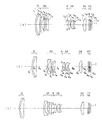•••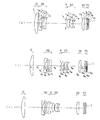•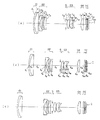•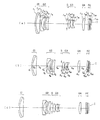•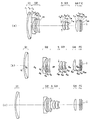•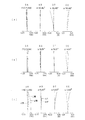•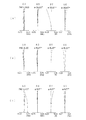•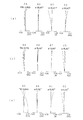•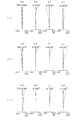•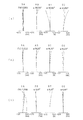•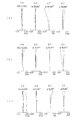•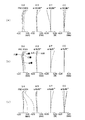•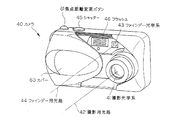•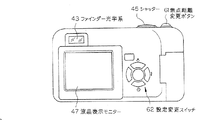•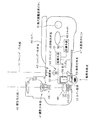## Abstract

PROBLEM TO BE SOLVED: To provide a zoom lens which is compact, bright and easy to achieve a high zoom ratio, and an imaging apparatus equipped with the zoom lens.
SOLUTION: A positive first group G1, a negative second group G2, a positive third group G3, and a positive fourth group G4 have a distance at the telephoto end with respect to the wide angle end during zooming. The first group G1 and the second group G2 are enlarged, the second group G2 and the third group G3 are reduced, and the third group G3 and the fourth group G4 are enlarged. The first group G1, the second group G2, and the third group G3 move on the optical axis. The first group G1 includes at most two lenses. The second group G2 includes a negative lens L21 and a negative lens L22. A zoom lens that includes a positive lens L23 and satisfies a conditional expression that specifies an appropriate refractive power of the third lens group G3.
[Selection] Figure 1

## Description

The present invention relates to a zoom lens and an image pickup apparatus including the zoom lens, and more particularly to a zoom lens having a zoom ratio of 4 times or more suitable for an electronic image pickup device such as a CCD and an image pickup apparatus including the zoom lens.

In recent years, digital cameras have become widespread. Furthermore, it has come to have a number of categories in a wide range from a high-function type for business use to a portable popular type. In the present invention, particularly focusing on the category of portable popular types, aiming to provide a technology for realizing a video camera and a digital camera with a relatively high zoom ratio and a thin depth while ensuring high image quality. Yes.

The biggest bottleneck in reducing the depth direction of the camera is the thickness from the most object-side surface to the imaging surface of the optical system, particularly the zoom lens system. Recently, it has become a mainstream to employ a so-called collapsible lens barrel that projects an optical system from the camera body during shooting and stores the optical system in the camera body when carried.

In order to reduce the thickness and size of the image sensor, it is only necessary to reduce the size of the image sensor. However, in order to obtain the same number of pixels, it is necessary to reduce the pixel pitch, and the lack of sensitivity must be covered by the optical system. The same is true for diffraction. Therefore, an optical system with a bright F value is required. As such an optical system, in order from the object side, a first group having a positive refractive power, a second group having a negative refractive power, a third group having a positive refractive power, and a positive refractive power. There is known an optical system that includes four lens groups of the fourth group and has an interval between the lens groups during zooming. As conventional techniques of such an optical system, Patent Document 1, Patent Document 2, Patent Document 3, Patent Document 4, and Patent Document 5 are suitable for an electronic imaging device having a zoom ratio of about 4 to 5 times. Although a zoom lens with a small number of constituent lenses is disclosed, there are problems such as a long overall length and insufficient compactness. Further, Patent Document 6, Patent Document 7, Patent Document 8, and Patent Document 9 disclose such lens systems as conventional examples having a zoom ratio of about 3 times. Further, Patent Document 10 discloses an optical system suitable for a silver salt film having a zoom ratio of about 3. However, there is a problem that the F value is as dark as about 3.5 at the wide angle end.
JP 2003-315676 A JP 2003-43357 A JP 2001-42215 A JP 2004-12439 A JP 2004-12638 A JP 2001-356269 A JP 2001-242379 A JP 2001-194586 A JP 2000-188170 A JP-A-57-5012

The present invention has been made in view of such problems of the prior art, and an object of the present invention is to provide a zoom lens that is compact, bright and easy to increase the zoom ratio, and an image pickup apparatus equipped with the zoom lens. is there. More specifically, it is easy to reduce the number of components, the F-number is as bright as about 2.8 at the wide-angle end, the zoom ratio is as large as about 4 to 5 times, and a compact zoom lens with high imaging performance and it is installed. An object of the present invention is to provide an imaging apparatus.

In order to achieve the above object, a first zoom lens of the present invention includes, in order from the object side, a first lens group G1 having a positive refractive power, a second lens group G2 having a negative refractive power, and a positive The zoom lens has a third lens group G3 having a refractive power and a fourth lens group G4 having a positive refractive power, and at the time of zooming, the distance from the wide-angle end to the telephoto end is the first lens group G1 and the second lens group G2. Between the second lens group G2 and the third lens group G3, and the distance between the third lens group G3 and the fourth lens group G4 is expanded. The second lens group G2 and the third lens group G3 move on the optical axis, the first lens group G1 is composed of at most two lenses, and the second lens group G2 is a negative lens L21 in order from the object side. The negative lens L22 and the positive lens L23 satisfy the following conditional expression: And it is characterized in and.

1.2 <f 3 / f W <1.85 (1)
Where f W is the focal length of the entire system at the wide-angle end,
f 3 : focal length of the third lens group,
It is.

Hereinafter, the reason and effect | action which take the said structure in a 1st zoom lens are demonstrated.

By adopting a configuration in which the refractive powers of the lens units are arranged in order of positive, negative and positive in order from the object side, the performance can be maintained even when the zoom ratio exceeds four times.

During zooming, the distance between the first lens group G1 and the second lens group G2 is enlarged, and the distance between the second lens group G2 and the third lens group G3 is reduced, and the third lens is separated from the wide angle end at the telephoto end. By enlarging the space between the group G3 and the fourth lens group G4, it is possible to share the burden of zooming between the second lens group G2 and the third lens group G3 and to perform efficient zooming. Furthermore, by moving the first lens group G1 in addition to the second lens group G2 and the third lens group G3, it becomes easy to increase the zoom ratio with a compact optical system.

In addition, the first lens group G1 is composed of at most two lenses, so that the lens diameter of the first lens group G1 can be made compact. As a result, the thickness of the first lens group G1 can be reduced.

The second lens group G2 includes, in order from the object side, a negative lens L21, a negative lens L22, and a positive lens L23, so that an optical axis of the second lens group G2 is obtained while obtaining a zoom ratio and having power. By reducing the thickness in the direction, a space for zooming can be secured.

Furthermore, conditional expression (1) specifies an appropriate refractive power of the third lens group G3. When the upper limit of 1.85 in conditional expression (1) is exceeded, the zooming action in the third lens group G3 becomes weak, and the total length when zooming to the telephoto end becomes long. In addition, when the aperture stop is moved together with the third lens group G3, the variation amount of the exit pupil position becomes large, so that the incident angle fluctuation to the image pickup device such as a CCD becomes large at the off-axis image plane position, and shading is performed. Adversely affected. If the lower limit of the conditional expression (1) is less than 1.2, the number of aberrations increases and it becomes difficult to obtain good imaging performance unless the number of sheets is increased and the compactness is not impaired. In addition, it is difficult to obtain sufficient back focus.

In addition, the lower limit value of conditional expression (1) may be 1.4, or 1.6.

In addition, the upper limit value of conditional expression (1) may be set to 1.83, or even 1.81.

Furthermore, it is desirable that the first lens group G1 is positioned on the object side at the telephoto end with respect to the wide angle end. By doing so, the total length at the wide-angle end can be reduced, and it is advantageous for increasing the focal length at the telephoto end.

Alternatively, it is desirable that the second lens group G2 be closest to the image side between the wide-angle end and the telephoto end. That is, it is preferable that the second lens group G2 is moved along the locus convex toward the image side between the wide-angle end and the telephoto end. By doing so, the zooming burden is borne on the wide-angle side, while the moving range of the third lens group is secured on the telephoto side, which is advantageous for increasing the zooming ratio.

Alternatively, it is desirable that the third lens group G3 is moved to the object side substantially monotonically from the wide angle end to the telephoto end. That is, it is preferable that the third lens group G3 moves only to the object side during zooming from the wide-angle end to the telephoto end.

Further, the first lens group G1, the second lens group G2, and the third lens group G3 described above move as described above, so that the third lens group G3 in the wide angle range and the second lens group G2 in the telephoto range. The burden of zooming becomes large, and it becomes easy to ensure imaging performance even at high zooming.

The first lens group G1 is preferably composed of a single positive lens. This is preferable for shortening the overall length and reducing the cost of the first lens group G1.

Alternatively, it is desirable that the first lens group G1 is composed of one negative lens and one positive lens in order from the object side. By doing so, it is effective in correcting chromatic aberration and the like in the first lens group G1.

Furthermore, it is desirable that the first lens group G1 is composed of a cemented lens including one negative lens and one positive lens. By doing so, the reflected light between the two lens surfaces is suppressed, and the ghost is easily reduced.

The second zoom lens according to the present invention is characterized in that, in the first zoom lens, the third lens group G3 includes two positive lenses and one negative lens.

The reason and action of the second zoom lens having the above-described configuration will be described. With such a configuration, the third lens group G3 has a converging function, and the aberration of the third lens group G3 can be suppressed satisfactorily. Can be made thinner.

The third zoom lens of the present invention is characterized in that the following conditional expressions are satisfied in the first and second zoom lenses.

5.8 <f 1 / f W <8.0 (2)
Where f W is the focal length of the entire system at the wide-angle end,
f 1 : focal length of the first lens group,
It is.

Hereinafter, the reason and effect | action which take the said structure in a 3rd zoom lens are demonstrated.

Conditional expression (2) is a condition that appropriately determines the refractive power of the first lens group G1. If f 1 increases beyond the upper limit of 8.0 in conditional expression (2), it becomes difficult to perform zooming in the rear group while maintaining miniaturization. When the lower limit of 5.8 is exceeded, the amount of aberration generated in the first lens group G1 increases, making it difficult to obtain good imaging performance.

Further, the lower limit value of conditional expression (2) may be 5.95, or even 6.05.

In addition, the upper limit value of conditional expression (2) may be 7.5, or 7.0.

The fourth zoom lens of the present invention is characterized in that, in the first to third zoom lenses, the following conditional expression is satisfied.

2 <D 2W / D 3W <2.6 (3)
Where D 2W is the distance between the second lens group and the third lens group at the wide-angle end,
D 3W : Third lens group-fourth lens group spacing at the wide-angle end,
It is.

Hereinafter, the reason and effect | action which take the said structure in a 4th zoom lens are demonstrated.

Conditional expression (3) defines an appropriate group spacing ratio of the second lens group G2, the third lens group G3, and the fourth lens group G4 at the wide angle end. When the value exceeds the upper limit of 2.6 in the conditional expression (3), the first lens group G1 and the second lens group G2 at the wide angle are closer to the object side, the height of off-axis rays is increased, and the lens diameter is increased. It becomes easy to invite. Alternatively, when the aperture stop is moved together with the third lens group G3, the angle between the second lens group G2 and the third lens group G3 is narrowed, so that the exit angle to the image surface is likely to increase, resulting in deterioration of shading characteristics. . When the value is smaller than the lower limit 2 of the conditional expression (3), the distance between the second lens group G2 and the third lens group G3 becomes small, and it becomes difficult to secure a space necessary for zooming.

Further, the lower limit value of conditional expression (3) may be set to 2.05, and further 2.07.

Further, the upper limit value of conditional expression (3) may be set to 2.55, and further to 2.52.

The fifth zoom lens of the present invention is characterized in that, in the first to fourth zoom lenses, the following conditional expression is satisfied.

3 <(β 2T / β 2W ) * (β 3T / β 3W ) * (β 4T / β 4W ) <12 (4)
1.8 <D 2W / f W <2.8 (5)
Where β 2T , β 3T , β 4T : magnifications at the telephoto end of the second lens group, the third lens group, and the fourth lens group,
β 2W , β 3W , β 4W : magnifications at the wide angle end of the second lens group, the third lens group, and the fourth lens group,
f W : focal length of the entire system at the wide-angle end,
D 2W : second lens group-third lens group spacing at the wide-angle end,
It is.

Hereinafter, the reason and effect | action which take the said structure in a 5th zoom lens are demonstrated.

Conditional expression (4) relates to a basic zoom ratio. When the conditional expression (4) is satisfied and the upper limit of 2.8 of the conditional expression (5) is exceeded, the first lens group G1 and the second lens group G2 at the wide angle are close to the object side, and the height of off-axis rays is increased. Increases the lens diameter, or the distance between the second lens group G2 and the third lens group G3 is narrowed, resulting in an increase in the angle of emission to the image plane, resulting in deterioration of shading characteristics. If the lower limit of 1.8 of the conditional expression (5) is exceeded, the amount of change in the distance between the second lens group G2 and the third lens group G3 cannot be ensured, and conditional expression (4) is ensured while ensuring imaging performance. It becomes difficult to satisfy.

One or both of conditional expressions (4) and (5) may be in the following numerical ranges.

3 <(β 2T / β 2W ) * (β 3T / β 3W ) * (β 4T / β 4W ) <7 (4) ′
2.0 <D 2W / f W <2.8 (5) ′
Further, the lower limit value of conditional expression (4) may be 3.3, or 3.5.

Further, the upper limit value of conditional expression (4) may be set to 7.0, or even 6.3.

In addition, the lower limit value of conditional expression (5) may be set to 2.2, or even 2.35.

Further, the upper limit value of conditional expression (5) may be 2.75, or 2.7.

The sixth zoom lens of the present invention is characterized in that, in the first to fifth zoom lenses, the following conditional expression is satisfied.

0.8 <Δ T1G / Δ T3G <1.3 (6)
Where Δ T1G is the amount of movement of the first lens unit between the wide-angle end and the telephoto end,
Δ T3G : the amount of movement of the third lens group from the wide-angle end to the telephoto end,
It is.

Hereinafter, the reason and effect | action which take the said structure in a 6th zoom lens are demonstrated.

When the upper limit of 1.3 of conditional expression (6) is exceeded, the amount of movement of the first lens group G1 due to zooming increases, and the peripheral light amount at the telephoto end decreases unless the diameter of the first lens group G1 is increased. Becomes prominent. If the lower limit of 0.8 is exceeded, the zooming burden on the third lens group G3 increases, and the fluctuation of the open F-number increases. When the aperture is fixed, the F-number at the telephoto end increases and becomes dark. End up. Or, when the open aperture diameter is changed by zooming, the mechanism becomes complicated or the space efficiency becomes worse. If the effective diameter of the first lens group G1 is set at the wide-angle end, the entrance pupil position at the wide-angle end becomes deep, and the periphery of the first lens group G1 is not used at the telephoto end. Use efficiency is poor, and it is disadvantageous for downsizing.

Further, the lower limit value of conditional expression (6) may be set to 0.85, further 0.9.

In addition, the upper limit value of conditional expression (6) may be 1.25, or 1.2.

The seventh zoom lens of the present invention is characterized in that, in the sixth zoom lens, the following conditional expression is satisfied.

-0.20 <Δ S1G / Δ S3G <0.8 (7)
Where Δ S1G is the amount of movement of the first lens unit between the wide-angle end and the intermediate focal length state,
Δ T3G : the amount of movement of the third lens unit between the wide-angle end and the intermediate focal length state,
It is. However, the intermediate focal length state is a focal length f S represented by f S = √ (f W · f T ) when the zoom lens has a wide-angle end focal length f W and a telephoto end focal length f T. State.

Hereinafter, the reason and effect | action which take the said structure in a 7th zoom lens are demonstrated.

If the upper limit of 0.8 of conditional expression (7) is exceeded, the entrance pupil position becomes deeper in the intermediate region, and the incident light height of the first lens group G1 becomes higher, so the lens diameter of the first lens group G1 becomes larger. Increase. If the lower limit of −0.20 is exceeded, the amount of movement of the first lens group G1 from the intermediate focal length to the telephoto end becomes too large, which increases the mechanical burden.

In addition, the lower limit value of conditional expression (7) may be set to −0.1, further 0, and further 0.1.

In addition, the upper limit value of conditional expression (7) may be set to 0.75, or 0.7.

The eighth zoom lens of the present invention is the first to seventh zoom lenses, and the second lens group G2 includes, in order from the object side, a negative meniscus lens L21, a negative meniscus lens or a plano-concave negative lens L22, and a positive meniscus lens. It consists of L23, and satisfies the following conditional expressions.

3 <(β 2T / β 2W ) * (β 3T / β 3W ) * (β 4T / β 4W ) <12 (4)
1.1 <| f 2 / f W | <1.8 (8)
Where f W is the focal length of the entire system at the wide-angle end,
f 2 : focal length of the second lens group,
β 2T , β 3T , β 4T : magnifications at the telephoto end of the second lens group, the third lens group, and the fourth lens group,
β 2W , β 3W , β 4W : magnifications at the wide angle end of the second lens group, the third lens group, and the fourth lens group,
It is.

Hereinafter, the reason and effect of the above configuration in the eighth zoom lens will be described.

The second lens group G2 includes, in order from the object side, a negative meniscus lens L21, a negative meniscus lens or a plano-concave negative lens L22, and a positive meniscus lens L23, so that high-order aberrations can be accurately controlled. Ensuring performance is easy.

Furthermore, conditional expression (4) relates to the basic zoom ratio. When the conditional expression (4) is satisfied and the upper limit of 1.8 to the conditional expression (8) is exceeded, it is difficult to obtain a zoom ratio defined by the conditional expression (4) with a compact size. If the lower limit of 1.1 is exceeded, it will be difficult to ensure power with a negative meniscus lens or plano-concave negative lens alone.

Further, the lower limit value of conditional expression (4) may be 3.3, or 3.5.

Further, the upper limit value of conditional expression (4) may be set to 7.0, or even 6.3.

In addition, the lower limit value of conditional expression (8) may be set to 1.3, or even 1.35.

In addition, the upper limit value of conditional expression (8) may be 1.7, or 1.6.

According to a ninth zoom lens of the present invention, in the first to eighth zoom lenses, the refractive indexes of the glass materials of the two negative lenses in the second lens group G2 are both 1.81 or more, and the second lens The refractive index of the glass material of the positive lens in group G2 is 1.9 or more.

The reason and action of the ninth zoom lens having the above configuration will be described. The refractive indexes of the glass materials of the two negative lenses in the second lens group G2 are both 1.81 or less, and the second lens group G2. If the refractive index of the glass material of the positive lens is 1.9 or less, it is difficult to correct chromatic aberration. In addition, the Petzval sum increases in the negative direction, and the field curvature increases.

The tenth zoom lens of the present invention is characterized in that, in the first to ninth zoom lenses, the following conditional expression is satisfied.

6.4 <L W / f W <7.4 (9)
Where L W is the total length at the wide-angle end,
f W : focal length of the entire system at the wide-angle end,
It is.

Hereinafter, the reason and action of the tenth zoom lens having the above configuration will be described.

Conditional expression (9) defines the ratio of the total length (length from the first surface of the lens system to the image plane) with respect to the focal length of the entire system at the wide-angle end, and when the upper limit of 7.4 is exceeded, The overall length at the wide-angle end becomes longer, leading to an increase in the size of the lens unit. In addition, since the height of the light beam in the first lens group G1 increases, the lens diameter increases. If the lower limit of 6.4 is exceeded, as a result, the distance between the second lens group G2 and the third lens group G3 becomes narrow, and it becomes impossible to secure a space necessary for zooming.

In addition, the lower limit value of conditional expression (9) may be set to 6.55, or 6.5.

Also, the upper limit value of conditional expression (9) may be set to 7.2, or 7.0.

In the present invention described above, it is preferable that the fourth lens group G4 includes a single lens from the viewpoint of compactness including the drive mechanism. Further, focusing is preferably performed by the fourth lens group G4. In addition, it is preferable that the aperture stop is disposed on the object side of the third lens group G3 and moves integrally with the third lens group G3. The third lens group G3 includes, in order from the object side, a biconvex lens L31, a positive meniscus lens L32 having a concave surface facing the image surface side, and a negative meniscus lens L33 having a concave surface facing the image surface side. Is preferred. The positive meniscus lens L32 and the negative meniscus lens L33 are preferably cemented lenses.

In order to make the present invention compact, it is preferable to have a four-group configuration. However, a lens group with low power may be added, and while enjoying the effects of the present invention, the lens group on the image plane side. May be added to make a zoom lens with higher magnification, higher performance, or expanded functions.

The image pickup apparatus of the present invention includes the zoom lens as described above and an image pickup element arranged on the image side thereof. The zoom lens is compact, bright and has a high zoom ratio. Therefore, if such a zoom lens is mounted on an imaging apparatus as an imaging optical system, it is possible to reduce the size and increase the functionality. In addition to the digital camera, the imaging device includes a video camera, a digital video unit, and the like.

According to the zoom lens of the present invention and the image pickup apparatus equipped with the zoom lens, it is possible to obtain a zoom lens that is compact, bright and easy to achieve a high zoom ratio, and an image pickup apparatus equipped with the zoom lens. It is easy to reduce the number of images, and it is possible to obtain a compact zoom lens having a high F-number of about 2.8 at the wide-angle end, a large zoom ratio of about 4 to 5 times, and a high imaging performance, and an image pickup apparatus equipped with the zoom lens. it can.

Examples 1 to 7 of the zoom lens according to the present invention will be described below. FIGS. 1 to 7 show lens cross-sectional views at the wide-angle end (a), the intermediate state (b), and the telephoto end (c) when focusing on an object point at infinity in Examples 1 to 7, respectively. In the figure, the first lens group is G1, the second lens group is G2, the aperture stop is S, the third lens group is G3, the fourth lens group is G4, and the parallel plates constituting the low-pass filter with IR cut coating are F, C is the parallel plate of the cover glass of the electronic image sensor, and I is the image plane. In addition, you may give the multilayer film for wavelength range limitation to the surface of the cover glass CG. Further, the cover glass CG may have a low-pass filter function.

As shown in FIG. 1, the zoom optical system according to the first embodiment includes, in order from the object side, a first lens group G1 having a positive refractive power, a second lens group G2 having a negative refractive power, an aperture stop S, a positive Is composed of a third lens group G3 having a refractive power of 4 and a fourth lens group G4 having a positive refractive power. When zooming from the wide-angle end to the telephoto end, the first lens group G1 The second lens group G2 moves along a convex locus on the image side, and is located closer to the image side at the telephoto end than at the wide-angle end. The aperture stop S and the third lens group G3 integrally move monotonically toward the object side, and the fourth lens group G4 moves along a locus convex toward the object side, and is positioned closer to the object side than the wide-angle end position at the telephoto end. To do.

In order from the object side, the first lens group G1 includes a cemented lens of a negative meniscus lens having a convex surface facing the object side and a positive meniscus lens having a convex surface facing the object side, and the second lens group G2 has a convex surface facing the object side. A negative meniscus lens having a convex surface, a plano-concave negative lens, and a positive meniscus lens having a convex surface facing the object side. The third lens group G3 includes a biconvex positive lens and a positive meniscus having a convex surface facing the object side. The fourth lens group G4 includes a single biconvex positive lens. The fourth lens group G4 includes a lens and a cemented lens of a negative meniscus lens having a convex surface facing the object side.

The aspheric surfaces are used on both surfaces of the biconvex positive lens of the third lens group G3 and both surfaces of the biconvex positive lens of the fourth lens group G4.

As shown in FIG. 2, the zoom optical system according to the second embodiment includes, in order from the object side, a first lens group G1 having a positive refractive power, a second lens group G2 having a negative refractive power, an aperture stop S, a positive Is composed of a third lens group G3 having a refractive power of 4 and a fourth lens group G4 having a positive refractive power. When zooming from the wide-angle end to the telephoto end, the first lens group G1 The second lens group G2 moves along a convex locus on the image side, and is located closer to the image side at the telephoto end than at the wide-angle end. The aperture stop S and the third lens group G3 integrally move monotonically toward the object side, and the fourth lens group G4 moves along a locus convex toward the object side, and is positioned closer to the object side than the wide-angle end position at the telephoto end. To do.

In order from the object side, the first lens group G1 is composed of a cemented lens of a negative meniscus lens having a convex surface facing the object side and a biconvex positive lens, and the second lens group G2 is a negative meniscus lens having a convex surface facing the object side. The third lens group G3 includes a biconvex positive lens, a positive meniscus lens having a convex surface on the object side, and a negative meniscus having a convex surface on the object side. The fourth lens group G4 is composed of a single biconvex positive lens.

Aspherical surfaces are used on both surfaces of the biconvex positive lens of the third lens group G3.

As shown in FIG. 3, the zoom optical system according to the third embodiment includes, in order from the object side, a first lens group G1 having a positive refractive power, a second lens group G2 having a negative refractive power, an aperture stop S, a positive Is composed of a third lens group G3 having a refractive power of 4 and a fourth lens group G4 having a positive refractive power. When zooming from the wide-angle end to the telephoto end, the first lens group G1 The second lens group G2 moves along a convex locus on the image side, and is located closer to the image side at the telephoto end than at the wide-angle end. The aperture stop S and the third lens group G3 integrally move monotonically toward the object side, and the fourth lens group G4 moves along a locus convex toward the object side, and is positioned closer to the object side than the wide-angle end position at the telephoto end. To do.

In order from the object side, the first lens group G1 is composed of a cemented lens of a negative meniscus lens having a convex surface facing the object side and a biconvex positive lens, and the second lens group G2 is a negative meniscus lens having a convex surface facing the object side. The third lens group G3 includes a biconvex positive lens, a positive meniscus lens having a convex surface on the object side, and a negative meniscus having a convex surface on the object side. The fourth lens group G4 is composed of a single biconvex positive lens.

Aspherical surfaces are used on both surfaces of the biconvex positive lens of the third lens group G3.

As shown in FIG. 4, the zoom optical system according to the fourth embodiment includes, in order from the object side, a first lens group G1 having a positive refractive power, a second lens group G2 having a negative refractive power, an aperture stop S, a positive Is composed of a third lens group G3 having a refractive power of 4 and a fourth lens group G4 having a positive refractive power. When zooming from the wide-angle end to the telephoto end, the first lens group G1 The second lens group G2 moves along a convex locus on the image side, and is located closer to the image side at the telephoto end than at the wide-angle end. The aperture stop S and the third lens group G3 integrally move monotonically toward the object side, and the fourth lens group G4 moves toward the object side while increasing the distance from the third lens group G3.

In order from the object side, the first lens group G1 includes one biconvex positive lens, and the second lens group G2 includes two negative meniscus lenses having a convex surface on the object side and a positive surface having a convex surface on the object side. The third lens group G3 includes a biconvex positive lens, a cemented lens of a positive meniscus lens having a convex surface facing the object side, and a negative meniscus lens having a convex surface facing the object side. The group G4 is composed of one biconvex positive lens.

The aspheric surfaces are used for the three surfaces of the biconvex positive lens of the third lens group G3 and the object side surface of the biconvex positive lens of the fourth lens group G4.

As shown in FIG. 5, the zoom optical system according to the fifth embodiment includes, in order from the object side, a first lens group G1 having a positive refractive power, a second lens group G2 having a negative refractive power, an aperture stop S, a positive Is composed of a third lens group G3 having a refractive power of 4 and a fourth lens group G4 having a positive refractive power. When zooming from the wide-angle end to the telephoto end, the first lens group G1 The second lens group G2 moves along a convex locus on the image side, and is located closer to the image side at the telephoto end than at the wide-angle end. The aperture stop S and the third lens group G3 integrally move monotonically toward the object side, and the fourth lens group G4 moves along a locus convex toward the object side, and is positioned closer to the object side than the wide-angle end position at the telephoto end. To do.

In order from the object side, the first lens group G1 is composed of a cemented lens of a negative meniscus lens having a convex surface facing the object side and a biconvex positive lens, and the second lens group G2 is a negative meniscus lens having a convex surface facing the object side. The third lens group G3 includes a biconvex positive lens, a positive meniscus lens having a convex surface on the object side, and a negative meniscus having a convex surface on the object side. The fourth lens group G4 is composed of a single biconvex positive lens.

The aspheric surfaces are used for the three surfaces of the biconvex positive lens of the third lens group G3 and the object side surface of the biconvex positive lens of the fourth lens group G4.

As shown in FIG. 6, the zoom optical system according to the sixth embodiment includes, in order from the object side, a first lens group G1 having a positive refractive power, a second lens group G2 having a negative refractive power, an aperture stop S, a positive Is composed of a third lens group G3 having a refractive power of 4 and a fourth lens group G4 having a positive refractive power. When zooming from the wide-angle end to the telephoto end, the first lens group G1 The second lens group G2 moves along a convex locus on the image side, and is located closer to the image side at the telephoto end than at the wide-angle end. The aperture stop S and the third lens group G3 integrally move monotonically toward the object side, and the fourth lens group G4 moves along a locus convex toward the object side, and is positioned closer to the object side than the wide-angle end position at the telephoto end. To do.

In order from the object side, the first lens group G1 includes a cemented lens of a negative meniscus lens having a convex surface facing the object side and a positive meniscus lens having a convex surface facing the object side, and the second lens group G2 has a convex surface facing the object side. A negative meniscus lens having a convex surface, a plano-concave negative lens, and a positive meniscus lens having a convex surface facing the object side. The third lens group G3 includes a biconvex positive lens and a positive meniscus having a convex surface facing the object side. The fourth lens group G4 is composed of one positive meniscus lens having a convex surface facing the object side and a cemented lens of a negative meniscus lens having a convex surface facing the object side.

The aspheric surfaces are used on the four surfaces of the biconvex positive lens of the third lens group G3 and the both surfaces of the positive meniscus lens of the fourth lens group G4.

As shown in FIG. 7, the zoom optical system according to the seventh embodiment includes, in order from the object side, a first lens group G1 having a positive refractive power, a second lens group G2 having a negative refractive power, an aperture stop S, a positive Is composed of a third lens group G3 having a refractive power of 4 and a fourth lens group G4 having a positive refractive power. When zooming from the wide-angle end to the telephoto end, the first lens group G1 The second lens group G2 moves to the image side. The aperture stop S and the third lens group G3 integrally move monotonically toward the object side, and the fourth lens group G4 moves along a locus convex toward the object side, and is positioned closer to the object side than the wide-angle end position at the telephoto end. To do.

In order from the object side, the first lens group G1 is composed of a cemented lens of a negative meniscus lens having a convex surface facing the object side and a biconvex positive lens, and the second lens group G2 is a negative meniscus lens having a convex surface facing the object side. The third lens group G3 includes a biconvex positive lens, a positive meniscus lens having a convex surface on the object side, and a negative meniscus having a convex surface on the object side. The fourth lens group G4 is composed of a single biconvex positive lens.

Aspherical surfaces are used on both surfaces of the biconvex positive lens of the third lens group G3.

The numerical data of each of the above embodiments are shown below. Symbols are the above, f is the total focal length, FNO is the F number, ω is the half angle of view, WE is the wide angle end, ST is the intermediate state, TE telephoto end, r 1, r 2 ... curvature radius of each lens surface, d 1, d 2 ... the spacing between the lens surfaces, n d1, n d2 ... d-line refractive index of each lens, [nu d1 , Ν d2 ... Is the Abbe number of each lens. The aspherical shape is represented by the following formula, where x is an optical axis with the light traveling direction being positive, and y is a direction orthogonal to the optical axis.

x = (y 2 / r) / [1+ {1- (K + 1) (y / r) 2 } 1/2 ]
+ A 4 y 4 + A 6 y 6 + A 8 y 8 + A 10 y 10 + A 12 y 12 + A 14 y 14
Where r is the paraxial radius of curvature, K is the conic coefficient, A 4 , A 6 , A 8 , A 10 , A 12 , A 14 are 4th, 6th, 8th, 10th, 12th, 14th, respectively. Is the aspheric coefficient.

Example 1
r 1 = 26.052 d 1 = 1.00 n d1 = 1.84666 ν d1 = 23.78
r 2 = 18.749 d 2 = 3.20 n d2 = 1.71300 ν d2 = 53.87
r 3 = 129.413 d 3 = (variable)
r 4 = 44.225 d 4 = 0.90 n d3 = 1.88300 ν d3 = 40.76
r 5 = 8.187 d 5 = 2.50
r 6 = ∞ d 6 = 0.85 n d4 = 1.88300 ν d4 = 40.76
r 7 = 15.273 d 7 = 1.09
r 8 = 13.756 d 8 = 2.00 n d5 = 1.92286 ν d5 = 20.88
r 9 = 56.639 d 9 = (variable)
r 10 = ∞ (aperture) d 10 = 1.00
r 11 = 13.960 (aspherical surface) d 11 = 2.00 n d6 = 1.74330 ν d6 = 49.33
r 12 = -35.983 (aspherical surface) d 12 = 0.50
r 13 = 6.486 d 13 = 2.25 n d7 = 1.69680 ν d7 = 55.53
r 14 = 21.204 d 14 = 0.01 n d8 = 1.56384 ν d8 = 60.67
r 15 = 21.204 d 15 = 0.80 n d9 = 1.84666 ν d9 = 23.78
r 16 = 4.830 d 16 = (variable)
r 17 = 11.548 (aspherical surface) d 17 = 2.74 n d10 = 1.52542 ν d10 = 55.78
r 18 = 218.908 (aspherical surface) d 18 = (variable)
r 19 = ∞ d 19 = 0.95 n d11 = 1.54771 ν d11 = 62.84
r 20 = ∞ d 20 = 0.60
r 21 = ∞ d 21 = 0.50 n d12 = 1.51633 ν d12 = 64.14
r 22 = ∞ d 22 = 1.00
r 23 = ∞
Aspheric coefficient 11th surface K = -0.392
A 4 = -5.42519 × 10 -5
A 6 = -7.99687 × 10 -6
A 8 = 6.60675 × 10 -7
A 10 = -1.95317 × 10 -8
Surface 12 K = 0.000
A 4 = 4.18583 × 10 -6
A 6 = -9.94224 × 10 -6
A 8 = 8.61195 × 10 -7
A 10 = -2.49912 × 10 -8
Surface 17 K = 0.000
A 4 = -2.11365 × 10 -4
A 6 = -2.70713 × 10 -6
A 8 = 3.48360 × 10 -7
A 10 = -1.13679 × 10 -8
A 12 = -1.43708 × 10 -10
A 14 = 6.12273 × 10 -12
18th face K = 0.000
A 4 = -2.44874 × 10 -4
A 6 = 6.46769 × 10 -6
A 8 = -2.13764 × 10 -7
A 10 = -7.27999 × 10 -11
A 12 = 3.72890 × 10 -11
A 14 = -6.98251 × 10 -13
Zoom data (∞)
WE ST TE
f (mm) 8.026 17.475 38.830
F NO 2.88 3.34 4.39
ω (°) 30.46 14.51 6.43
d 3 0.80 10.39 19.17
d 9 18.16 6.64 1.67
d 16 8.63 10.11 19.99
d 18 2.68 5.70 3.48.

Example 2
r 1 = 29.488 d 1 = 1.00 n d1 = 1.84666 ν d1 = 23.78
r 2 = 19.156 d 2 = 3.60 n d2 = 1.67790 ν d2 = 50.72
r 3 = -390894.267 d 3 = (variable)
r 4 = 177.797 d 4 = 0.85 n d3 = 1.88300 ν d3 = 40.76
r 5 = 9.237 d 5 = 2.00
r 6 = 2214.408 d 6 = 0.80 n d4 = 1.72916 ν d4 = 54.68
r 7 = 14.895 d 7 = 1.20
r 8 = 13.788 d 8 = 2.20 n d5 = 1.84666 ν d5 = 23.78
r 9 = 60.326 d 9 = (variable)
r 10 = ∞ (aperture) d 10 = 1.00
r 11 = 10.975 (aspherical surface) d 11 = 2.30 n d6 = 1.69350 ν d6 = 53.20
r 12 = -33.341 (aspherical surface) d 12 = 0.10
r 13 = 5.712 d 13 = 2.50 n d7 = 1.49700 ν d7 = 81.54
r 14 = 10.692 d 14 = 0.01 n d8 = 1.56384 ν d8 = 60.67
r 15 = 10.692 d 15 = 0.80 n d9 = 1.84666 ν d9 = 23.78
r 16 = 4.300 d 16 = (variable)
r 17 = 11.921 d 17 = 2.20 n d10 = 1.48749 ν d10 = 70.23
r 18 = -10407.303 d 18 = (variable)
r 19 = ∞ d 19 = 0.95 n d11 = 1.54771 ν d11 = 62.84
r 20 = ∞ d 20 = 0.60
r 21 = ∞ d 21 = 0.50 n d12 = 1.51633 ν d12 = 64.14
r 22 = ∞ d 22 = 0.60
r 23 = ∞
Aspheric coefficient 11th surface K = -1.149
A 4 = -1.22308 × 10 -8
A 6 = 8.10316 × 10 -9
A 8 = -5.55681 × 10 -9
Surface 12 K = -10.109
A 4 = 3.71688 × 10 -11
A 6 = 2.51887 × 10 -7
Zoom data (∞)
WE ST TE
f (mm) 7.843 15.841 39.635
F NO 2.80 3.13 4.50
ω (°) 30.67 15.37 6.17
d 3 0.80 9.57 18.65
d 9 20.01 8.14 1.50
d 16 8.69 8.91 20.02
d 18 1.98 5.16 2.67.

Example 3
r 1 = 26.986 d 1 = 1.00 n d1 = 1.84666 ν d1 = 23.78
r 2 = 16.515 d 2 = 3.80 n d2 = 1.67003 ν d2 = 47.23
r 3 = -244257.033 d 3 = (variable)
r 4 = 112.654 d 4 = 0.85 n d3 = 1.88300 ν d3 = 40.76
r 5 = 9.347 d 5 = 2.00
r 6 = 18401.111 d 6 = 0.80 n d4 = 1.72916 ν d4 = 54.68
r 7 = 11.494 d 7 = 1.20
r 8 = 12.358 d 8 = 2.20 n d5 = 1.84666 ν d5 = 23.78
r 9 = 53.415 d 9 = (variable)
r 10 = ∞ (aperture) d 10 = 1.00
r 11 = 9.677 (aspherical surface) d 11 = 2.30 n d6 = 1.58313 ν d6 = 59.46
r 12 = -25.547 (aspherical surface) d 12 = 0.10
r 13 = 5.680 d 13 = 2.30 n d7 = 1.49700 ν d7 = 81.54
r 14 = 8.984 d 14 = 0.01 n d8 = 1.56384 ν d8 = 60.67
r 15 = 8.984 d 15 = 0.80 n d9 = 1.84666 ν d9 = 23.78
r 16 = 4.272 d 16 = (variable)
r 17 = 12.940 d 17 = 2.20 n d10 = 1.49700 ν d10 = 81.54
r 18 = -23190.917 d 18 = (variable)
r 19 = ∞ d 19 = 0.95 n d11 = 1.54771 ν d11 = 62.84
r 20 = ∞ d 20 = 0.60
r 21 = ∞ d 21 = 0.50 n d12 = 1.51633 ν d12 = 64.14
r 22 = ∞ d 22 = 0.59
r 23 = ∞
Aspheric coefficient 11th surface K = -1.239
A 4 = -6.46005 × 10 -9
A 6 = 1.85826 × 10 -8
A 8 = -2.59108 × 10 -8
Surface 12 K = -4.428
A 4 = 2.79009 × 10 -10
A 6 = 3.14257 × 10 -7
Zoom data (∞)
WE ST TE
f (mm) 7.853 15.765 45.985
F NO 2.80 3.18 4.84
ω (°) 30.47 15.46 5.32
d 3 0.80 8.80 19.39
d 9 20.04 8.14 1.50
d 16 9.33 8.60 22.68
d 18 2.21 6.37 2.35.

Example 4
r 1 = 25.729 d 1 = 3.30 n d1 = 1.48749 ν d1 = 70.23
r 2 = -195211.986 d 2 = (variable)
r 3 = 62.315 d 3 = 0.85 n d2 = 1.88300 ν d2 = 40.76
r 4 = 8.171 d 4 = 2.00
r 5 = 11064.958 d 5 = 0.80 n d3 = 1.72916 ν d3 = 54.68
r 6 = 16.294 d 6 = 1.15
r 7 = 13.261 d 7 = 2.20 n d4 = 1.84666 ν d4 = 23.78
r 8 = 54.781 d 8 = (variable)
r 9 = ∞ (aperture) d 9 = 1.00
r 10 = 11.449 (aspherical surface) d 10 = 2.40 n d5 = 1.69350 ν d5 = 53.20
r 11 = -24.146 (aspherical surface) d 11 = 0.10
r 12 = 5.500 d 12 = 2.60 n d6 = 1.48749 ν d6 = 70.23
r 13 = 18.893 d 13 = 0.01 n d7 = 1.56384 ν d7 = 60.67
r 14 = 18.893 d 14 = 0.80 n d8 = 1.84666 ν d8 = 23.78
r 15 = 4.313 d 15 = (variable)
r 16 = 19.027 (aspherical surface) d 16 = 2.20 n d9 = 1.84666 ν d9 = 23.78
r 17 = -12117.666 d 17 = (variable)
r 18 = ∞ d 18 = 0.95 n d10 = 1.54771 ν d10 = 62.84
r 19 = ∞ d 19 = 0.60
r 20 = ∞ d 20 = 0.50 n d11 = 1.51633 ν d11 = 64.14
r 21 = ∞ d 21 = 0.59
r 22 = ∞
Aspheric coefficient 10th surface K = -1.005
A 4 = 3.59202 × 10 -9
A 6 = 1.69640 × 10 -8
A 8 = -1.84829 × 10 -8
11th surface K = -8.581
A 4 = -2.03082 × 10 -11
A 6 = 4.48326 × 10 -10
16th surface K = 0.002
A 4 = -3.12266 × 10 -10
A 6 = 1.67936 × 10 -8
A 8 = 9.76314 × 10 -9
Zoom data (∞)
WE ST TE
f (mm) 7.823 15.672 31.181
F NO 2.80 3.28 4.28
ω (°) 30.41 15.32 7.89
d 2 0.80 10.32 16.18
d 8 19.10 8.70 1.81
d 15 8.45 10.63 17.69
d 17 1.94 3.58 4.37.

Example 5
r 1 = 33.302 d 1 = 1.00 n d1 = 1.84666 ν d1 = 23.78
r 2 = 19.902 d 2 = 3.60 n d2 = 1.71700 ν d2 = 47.92
r 3 = -10505.082 d 3 = (variable)
r 4 = 197.422 d 4 = 0.85 n d3 = 1.81600 ν d3 = 46.62
r 5 = 8.612 d 5 = 2.00
r 6 = 65.254 d 6 = 0.80 n d4 = 1.90366 ν d4 = 31.31
r 7 = 13.669 d 7 = 0.80
r 8 = 12.087 d 8 = 2.20 n d5 = 1.92286 ν d5 = 20.88
r 9 = 43.061 d 9 = (variable)
r 10 = ∞ (aperture) d 10 = 1.00
r 11 = 10.627 (aspherical surface) d 11 = 2.30 n d6 = 1.69350 ν d6 = 53.20
r 12 = -33.162 (aspherical surface) d 12 = 0.10
r 13 = 5.800 d 13 = 2.50 n d7 = 1.49700 ν d7 = 81.54
r 14 = 11.640 d 14 = 0.01 n d8 = 1.56384 ν d8 = 60.67
r 15 = 11.640 d 15 = 0.80 n d9 = 1.84666 ν d9 = 23.78
r 16 = 4.350 d 16 = (variable)
r 17 = 12.332 (aspherical surface) d 17 = 2.30 n d10 = 1.52542 ν d10 = 55.78
r 18 = -260808.000 d 18 = (variable)
r 19 = ∞ d 19 = 0.95 n d11 = 1.54771 ν d11 = 62.84
r 20 = ∞ d 20 = 0.60
r 21 = ∞ d 21 = 0.50 n d12 = 1.51633 ν d12 = 64.14
r 22 = ∞ d 22 = 1.00
r 23 = ∞
Aspheric coefficient 11th surface K = -0.806
A 4 = -1.99973 × 10 -9
A 6 = 1.19364 × 10 -8
A 8 = -3.01523 × 10 -8
Surface 12 K = -22.194
A 4 = -1.02289 × 10 -11
A 6 = 1.12572 × 10 -8
A 8 = -1.88817 × 10 -9
Surface 17 K = 0.000
A 4 = -4.79706 × 10 -13
A 6 = 8.43506 × 10 -14
A 8 = -2.26626 × 10 -12
A 10 = 1.27028 × 10 -10
Zoom data (∞)
WE ST TE
f (mm) 7.964 17.453 38.463
F NO 2.81 3.33 4.30
ω (°) 30.56 14.05 6.41
d 3 0.80 11.18 20.49
d 9 19.10 7.68 1.80
d 16 7.64 9.77 17.57
d 18 2.18 4.37 2.83.

Example 6
r 1 = 26.272 d 1 = 1.00 n d1 = 1.84666 ν d1 = 23.78
r 2 = 18.575 d 2 = 3.40 n d2 = 1.74100 ν d2 = 52.64
r 3 = 130.489 d 3 = (variable)
r 4 = 57.666 d 4 = 0.90 n d3 = 1.88300 ν d3 = 40.76
r 5 = 8.282 d 5 = 2.40
r 6 = ∞ d 6 = 0.85 n d4 = 1.88300 ν d4 = 40.76
r 7 = 15.434 d 7 = 0.99
r 8 = 13.647 d 8 = 2.30 n d5 = 1.92286 ν d5 = 20.88
r 9 = 60.518 d 9 = (variable)
r 10 = ∞ (aperture) d 10 = 1.00
r 11 = 14.164 (aspherical surface) d 11 = 2.00 n d6 = 1.74330 ν d6 = 49.33
r 12 = -33.166 (aspherical surface) d 12 = 0.20
r 13 = 5.685 d 13 = 2.20 n d7 = 1.58913 ν d7 = 61.14
r 14 = 16.732 d 14 = 0.01 n d8 = 1.56384 ν d8 = 60.67
r 15 = 16.732 d 15 = 0.80 n d9 = 1.84666 ν d9 = 23.78
r 16 = 4.631 d 16 = (variable)
r 17 = 15.194 d 17 = 2.00 n d10 = 1.74330 ν d10 = 49.33
r 18 = 149.038 (aspherical surface) d 18 = (variable)
r 19 = ∞ d 19 = 0.95 n d11 = 1.54771 ν d11 = 62.84
r 20 = ∞ d 20 = 0.60
r 21 = ∞ d 21 = 0.50 n d12 = 1.51633 ν d12 = 64.14
r 22 = ∞ d 22 = 1.00
r 23 = ∞
Aspheric coefficient 11th surface K = 1.826
A 4 = -1.43848 × 10 -4
A 6 = 3.29825 × 10 -7
A 8 = 1.52442 × 10 -7
Surface 12 K = 0.000
A 4 = 3.01488 × 10 -5
A 6 = 8.15030 × 10 -7
A 8 = 2.16546 × 10 -7
18th face K = 0.000
A 4 = 3.59845 × 10 -5
A 6 = -1.74722 × 10 -5
A 8 = 7.23073 × 10 -7
A 10 = -1.56839 × 10 -8
Zoom data (∞)
WE ST TE
f (mm) 8.048 17.491 38.757
F NO 2.88 3.29 4.32
ω (°) 30.66 14.47 6.46
d 3 0.80 10.10 18.34
d 9 18.15 6.59 1.71
d 16 8.62 9.60 19.76
d 18 3.58 6.73 4.40.

Example 7
r 1 = 46.354 d 1 = 1.00 n d1 = 1.84666 ν d1 = 23.78
r 2 = 29.847 d 2 = 3.71 n d2 = 1.72916 ν d2 = 54.68
r 3 = -435.042 d 3 = (variable)
r 4 = 132.877 d 4 = 0.85 n d3 = 1.88300 ν d3 = 40.76
r 5 = 9.672 d 5 = 2.00
r 6 = 44.500 d 6 = 0.80 n d4 = 1.72916 ν d4 = 54.68
r 7 = 15.195 d 7 = 1.20
r 8 = 12.581 d 8 = 2.20 n d5 = 1.84666 ν d5 = 23.78
r 9 = 30.232 d 9 = (variable)
r 10 = ∞ (aperture) d 10 = 1.00
r 11 = 8.374 (aspherical surface) d 11 = 2.30 n d6 = 1.69350 ν d6 = 53.20
r 12 = -50.760 (aspherical surface) d 12 = 0.10
r 13 = 6.725 d 13 = 2.50 n d7 = 1.49700 ν d7 = 81.54
r 14 = 13.421 d 14 = 0.01 n d8 = 1.56384 ν d8 = 60.67
r 15 = 13.421 d 15 = 0.80 n d9 = 1.84666 ν d9 = 23.78
r 16 = 4.300 d 16 = (variable)
r 17 = 13.192 d 17 = 2.25 n d10 = 1.51823 ν d10 = 58.90
r 18 = -135.470 d 18 = (variable)
r 19 = ∞ d 19 = 0.95 n d11 = 1.54771 ν d11 = 62.84
r 20 = ∞ d 20 = 0.60
r 21 = ∞ d 21 = 0.50 n d12 = 1.51633 ν d12 = 64.14
r 22 = ∞ d 22 = 0.94
r 23 = ∞
Aspheric coefficient 11th surface K = -1.4817
A 4 = 1.7947 × 10 -4
A 6 = 2.4308 × 10 -6
A 8 = -2.1403 × 10 -8
Surface 12 K = -16.8224
A 4 = 8.3493 × 10 -5
A 6 = 2.4324 × 10 -6
Zoom data (∞)
WE ST TE
f (mm) 7.68 17.00 37.44
F NO 2.80 3.16 4.22
ω (°) 30.96 14.15 6.49
d 3 0.80 14.60 24.11
d 9 23.29 9.33 1.50
d 16 7.67 8.18 16.13
d 18 1.56 4.11 3.27.

Aberration diagrams at the time of focusing on an object point at infinity in Examples 1 to 7 are shown in FIGS. In these aberration diagrams, (a) is a wide angle end, (b) is an intermediate state, (c) is a spherical aberration (SA), astigmatism (AS), distortion (DT), and lateral chromatic aberration at a telephoto end ( CC).

Values excluding the absolute value symbols in the conditional expressions (1) to (9) of Examples 1 to 7 are as follows. Conditional Example Example 1 Example 2 Example 3 Example 4 Example 5 Example 6 Example 7
1 1.81 1.77 1.78 1.74 1.72 1.82 1.847
2 6.12 6.36 6.10 6.75 6.59 5.82 8.16
3 2.10 2.30 2.15 2.26 2.50 2.10 3.16
4 4.84 5.05 5.86 3.99 4.83 4.82 4.88
5 2.39 2.68 2.68 2.57 2.52 2.38 3.16
6 1.15 0.95 1.00 0.84 1.22 1.09 1.15
7 0.57 0.09 -0.13 0.77 0.76 0.45 0.97
8 -1.42 -1.51 -1.41 -1.57 -1.53 -1.39 1.84
9 6.75 6.97 7.08 6.69 6.66 6.74 7.43
.

In Examples 1 to 7 above, focusing is performed by extending the fourth lens group G4 to the object side.

The zoom lens of the present invention as described above forms an object image, and the image is received by an image pickup device such as a CCD, and can be used for a photographing apparatus, particularly a digital camera or a video camera. The embodiment is illustrated below.

FIGS. 15 to 17 are conceptual diagrams of structures in which the zoom optical system according to the present invention is incorporated in the photographing optical system 41 of a digital camera. 15 is a front perspective view showing the appearance of the digital camera 40, FIG. 16 is a rear front view thereof, and FIG. 17 is a schematic perspective plan view showing the configuration of the digital camera 40. However, FIGS. 15 and 17 show a state in which the photographing optical system 41 is not retracted. In this example, the digital camera 40 includes a photographing optical system 41 having a photographing optical path 42, a finder optical system 43 having a finder optical path 44, a shutter 45, a flash 46, a liquid crystal display monitor 47, a focal length change button 61, a setting. When the photographing optical system 41 is retracted, including the change switch 62, the photographing optical system 41, the finder optical system 43 and the flash 46 are covered with the cover 60 by sliding the cover 60. Then, when the cover 60 is opened and the camera 40 is set to the photographing state, the photographing optical system 41 enters the non-collapsed state of FIG. 17, and when the shutter 45 disposed on the upper portion of the camera 40 is pressed, the photographing optical system is interlocked therewith. Photographing is performed through the system 41, for example, the zoom lens of the first embodiment. An object image formed by the photographing optical system 41 is formed on the imaging surface of the CCD 49 via a low-pass filter LF having an IR cut coat and a cover glass CG. The object image received by the CCD 49 is displayed as an electronic image on the liquid crystal display monitor 47 provided on the back of the camera via the processing means 51. Further, the processing means 51 is connected to a recording means 52 so that a photographed electronic image can be recorded. The recording means 52 may be provided separately from the processing means 51, or may be configured to perform recording / writing electronically using a floppy disk, memory card, MO, or the like. Further, it may be configured as a silver salt camera in which a silver salt film is arranged in place of the CCD 49.

Further, a finder objective optical system 53 is disposed on the finder optical path 44. The finder objective optical system 53 includes a plurality of lens groups (three groups in the figure) and two prisms, and includes a zoom optical system whose focal length changes in conjunction with the zoom lens of the photographing optical system 41. The object image formed by the finder objective optical system 53 is formed on the field frame 57 of the erecting prism 55 that is an image erecting member. Behind the erecting prism 55, an eyepiece optical system 59 for guiding the erect image to the observer eyeball E is disposed. A cover member 50 is disposed on the exit side of the eyepiece optical system 59.

In the digital camera 40 configured in this manner, the photographing optical system 41 has a high performance and a small size and can be retracted, so that a high performance and a small size can be realized.

FIG. 3 is a lens cross-sectional view of the zoom lens according to the first exemplary embodiment at the wide-angle end (a), the intermediate state (b), and the telephoto end (c) when focusing on an object point at infinity. FIG. 6 is a lens cross-sectional view similar to FIG. 1 of a zoom lens according to Embodiment 2. FIG. 6 is a lens cross-sectional view similar to FIG. 1 of a zoom lens according to Embodiment 3. FIG. 6 is a lens cross-sectional view similar to FIG. 1 illustrating a zoom lens according to a fourth exemplary embodiment. FIG. 6 is a lens cross-sectional view similar to FIG. 1 illustrating a zoom lens according to a fifth exemplary embodiment. FIG. 6 is a lens cross-sectional view similar to FIG. 1 illustrating a zoom lens according to Example 6; FIG. 10 is a lens cross-sectional view similar to FIG. 1 illustrating a zoom lens according to a seventh embodiment. FIG. 6 is an aberration diagram for Example 1 upon focusing on an object point at infinity. FIG. 6 is an aberration diagram for Example 2 upon focusing on an object point at infinity. FIG. 10 is an aberration diagram for Example 3 upon focusing on an object point at infinity. FIG. 10 is an aberration diagram for Example 4 upon focusing on an object point at infinity. FIG. 10 is an aberration diagram for Example 5 upon focusing on an object point at infinity. FIG. 10 is an aberration diagram for Example 6 upon focusing on an object point at infinity. FIG. 10 is an aberration diagram for Example 7 upon focusing on an object point at infinity. It is a front perspective view which shows the external appearance of the digital camera incorporating the zoom lens by this invention. FIG. 16 is a rear perspective view of the digital camera of FIG. 15. It is sectional drawing of the digital camera of FIG.

Explanation of symbols

G1 ... first lens group G2 ... second lens group G3 ... third lens group G4 ... fourth lens group S ... aperture stop F ... parallel plate C constituting a low-pass filter ... cover glass I ... image plane LF ... low-pass filter CG ... cover glass E ... observer eyeball 40 ... digital camera 41 ... photographing optical system 42 ... photographing optical path 43 ... finder optical system 44 ... finder optical path 45 ... shutter 46 ... flash 47 ... liquid crystal display monitor 49 ... CCD
DESCRIPTION OF SYMBOLS 50 ... Cover member 51 ... Processing means 52 ... Recording means 53 ... Viewfinder objective optical system 55 ... Erect prism 57 ... Field frame 59 ... Eyepiece optical system 60 ... Cover 61 ... Focal length change button 62 ... Setting change switch

## Claims (10)

1. In order from the object side, the first lens group G1 having a positive refractive power, the second lens group G2 having a negative refractive power, the third lens group G3 having a positive refractive power, and a positive refractive power. The zoom lens has a fourth lens group G4. During zooming, the distance between the first lens group G1 and the second lens group G2 is increased at the telephoto end with respect to the wide-angle end, and the second lens group G2 and the third lens group G3 are expanded. At least the first lens group G1, the second lens group G2, and the third lens group G3 move on the optical axis so that the distance between the third lens group G3 and the fourth lens group G4 increases. The first lens group G1 is composed of at most two lenses, and the second lens group G2 is composed of a negative lens L21, a negative lens L22, and a positive lens L23 in order from the object side. A zoom lens characterized by satisfaction.
1.2 <f 3 / f W <1.85 (1)
Where f W is the focal length of the entire system at the wide-angle end,
f 3 : focal length of the third lens group,
It is.
2. The zoom lens according to claim 1, wherein the third lens group G3 includes two positive lenses and one negative lens.
3. The zoom lens according to claim 1, wherein the following conditional expression is satisfied.
5.8 <f 1 / f W <8.0 (2)
Where f W is the focal length of the entire system at the wide-angle end,
f 1 : focal length of the first lens group,
It is.
4. The zoom lens according to any one of claims 1 to 3, wherein the following conditional expression is satisfied.
2 <D 2W / D 3W <2.6 (3)
Where D 2W is the distance between the second lens group and the third lens group at the wide-angle end,
D 3W : Third lens group-fourth lens group spacing at the wide-angle end,
It is.
5. 5. The zoom lens according to claim 1, wherein the following conditional expression is satisfied.
3 <(β 2T / β 2W ) * (β 3T / β 3W ) * (β 4T / β 4W ) <12 (4)
1.8 <D 2W / f W <2.8 (5)
Where β 2T , β 3T , β 4T : magnifications at the telephoto end of the second lens group, the third lens group, and the fourth lens group,
β 2W , β 3W , β 4W : magnifications at the wide angle end of the second lens group, the third lens group, and the fourth lens group,
f W : focal length of the entire system at the wide-angle end,
D 2W : second lens group-third lens group spacing at the wide-angle end,
It is.
6. The zoom lens according to claim 1, wherein the following conditional expression is satisfied.
0.8 <Δ T1G / Δ T3G <1.3 (6)
Where Δ T1G is the amount of movement of the first lens unit between the wide-angle end and the telephoto end,
Δ T3G : the amount of movement of the third lens group from the wide-angle end to the telephoto end,
It is.
7. The zoom lens according to claim 6, wherein the following conditional expression is satisfied.
-0.20 <Δ S1G / Δ S3G <0.8 (7)
Where Δ S1G is the amount of movement of the first lens unit between the wide-angle end and the intermediate focal length state,
Δ T3G : the amount of movement of the third lens unit between the wide-angle end and the intermediate focal length state,
It is. However, the intermediate focal length state is a focal length f S represented by f S = √ (f W · f T ) when the zoom lens has a wide-angle end focal length f W and a telephoto end focal length f T. State.
8. The second lens group G2 is composed of a negative meniscus lens L21, a negative meniscus lens or a plano-concave negative lens L22, and a positive meniscus lens L23 in order from the object side, and satisfies the following conditional expression: The zoom lens according to any one of 1 to 7.
3 <(β 2T / β 2W ) * (β 3T / β 3W ) * (β 4T / β 4W ) <12 (4)
1.1 <| f 2 / f W | <1.8 (8)
Where f W is the focal length of the entire system at the wide-angle end,
f 2 : focal length of the second lens group,
β 2T , β 3T , β 4T : magnifications at the telephoto end of the second lens group, the third lens group, and the fourth lens group,
β 2W , β 3W , β 4W : magnifications at the wide angle end of the second lens group, the third lens group, and the fourth lens group,
It is.
9. The refractive index of the glass material of the two negative lenses of the second lens group G2 is 1.81 or more, and the refractive index of the glass material of the positive lens of the second lens group G2 is 1.9 or more. The zoom lens according to any one of claims 1 to 8.
10. The zoom lens according to any one of claims 1 to 9, wherein the following conditional expression is satisfied.
6.4 <L W / f W <7.4 (9)
Where L W is the total length at the wide-angle end,
f W : focal length of the entire system at the wide-angle end,
It is.
JP2004201374A 2004-07-08 2004-07-08 Zoom lens and image pickup apparatus equipped with the same Active JP4605699B2 (en)

## Priority Applications (1)

Application Number Priority Date Filing Date Title
JP2004201374A JP4605699B2 (en) 2004-07-08 2004-07-08 Zoom lens and image pickup apparatus equipped with the same

## Applications Claiming Priority (2)

Application Number Priority Date Filing Date Title
JP2004201374A JP4605699B2 (en) 2004-07-08 2004-07-08 Zoom lens and image pickup apparatus equipped with the same
US11/114,039 US7283310B2 (en) 2004-04-30 2005-04-26 Zoom lens, and imaging system incorporating it

## Publications (3)

Publication Number Publication Date
JP2006023531A5 JP2006023531A5 (en) 2006-01-26
JP2006023531A true JP2006023531A (en) 2006-01-26
JP4605699B2 JP4605699B2 (en) 2011-01-05

# Family

## Family Applications (1)

Application Number Title Priority Date Filing Date
JP2004201374A Active JP4605699B2 (en) 2004-07-08 2004-07-08 Zoom lens and image pickup apparatus equipped with the same

## Country Status (1)

JP (1) JP4605699B2 (en)

## Cited By (8)

* Cited by examiner, † Cited by third party
Publication number Priority date Publication date Assignee Title
JP2006184416A (en) * 2004-12-27 2006-07-13 Konica Minolta Photo Imaging Inc Photographic optical system and imaging apparatus
JP2007212537A (en) * 2006-02-07 2007-08-23 Canon Inc Zoom lens and imaging apparatus having same
JP2008096924A (en) * 2006-10-16 2008-04-24 Ricoh Co Ltd Zoom lens, imaging apparatus and personal digital assistant device
JP2008112013A (en) * 2006-10-31 2008-05-15 Olympus Imaging Corp Wide-angle high variable power zoom lens and imaging apparatus using the same
US7433132B2 (en) 2006-11-15 2008-10-07 Olympus Imaging Corp. Zoom lens system and electronic image pickup apparatus using the same
JP2009251343A (en) * 2008-04-08 2009-10-29 Canon Inc Zoom lens and image pickup apparatus including the same
US7848029B2 (en) 2008-02-21 2010-12-07 Sony Corporation Retractable zoom lens
US8405917B2 (en) 2010-03-12 2013-03-26 Ricoh Company, Ltd. Zoom lens, imaging device and information device

## Citations (2)

* Cited by examiner, † Cited by third party
Publication number Priority date Publication date Assignee Title
JPH06160712A (en) * 1992-11-17 1994-06-07 Konica Corp Zoom lens
JP2004094233A (en) * 2002-08-13 2004-03-25 Pentax Corp Zoom lens system

## Patent Citations (2)

* Cited by examiner, † Cited by third party
Publication number Priority date Publication date Assignee Title
JPH06160712A (en) * 1992-11-17 1994-06-07 Konica Corp Zoom lens
JP2004094233A (en) * 2002-08-13 2004-03-25 Pentax Corp Zoom lens system

## Cited By (8)

* Cited by examiner, † Cited by third party
Publication number Priority date Publication date Assignee Title
JP2006184416A (en) * 2004-12-27 2006-07-13 Konica Minolta Photo Imaging Inc Photographic optical system and imaging apparatus
JP2007212537A (en) * 2006-02-07 2007-08-23 Canon Inc Zoom lens and imaging apparatus having same
JP2008096924A (en) * 2006-10-16 2008-04-24 Ricoh Co Ltd Zoom lens, imaging apparatus and personal digital assistant device
JP2008112013A (en) * 2006-10-31 2008-05-15 Olympus Imaging Corp Wide-angle high variable power zoom lens and imaging apparatus using the same
US7433132B2 (en) 2006-11-15 2008-10-07 Olympus Imaging Corp. Zoom lens system and electronic image pickup apparatus using the same
US7848029B2 (en) 2008-02-21 2010-12-07 Sony Corporation Retractable zoom lens
JP2009251343A (en) * 2008-04-08 2009-10-29 Canon Inc Zoom lens and image pickup apparatus including the same
US8405917B2 (en) 2010-03-12 2013-03-26 Ricoh Company, Ltd. Zoom lens, imaging device and information device

## Also Published As

Publication number Publication date
JP4605699B2 (en) 2011-01-05

## Similar Documents

Publication Publication Date Title
JP4612823B2 (en) Zoom lens and imaging apparatus having the same
JP4764644B2 (en) Zoom lens and information device
CN1162727C (en) Variable focus lens and optical apparatus with the same variable focus lens
US20050024749A1 (en) Zoom lens and optical apparatus having the same
DE10212171B4 (en) Zoom lens system
JP5117549B2 (en) Electronic imaging device
JP3943922B2 (en) Imaging device
JP2005266129A (en) Zoom lens and electronic imaging apparatus having the same
JP4354153B2 (en) Zoom lens, camera, and portable information terminal device
JP4938068B2 (en) Electronic imaging device
JP4153640B2 (en) Zoom lens and imaging device
CN102062935A (en) Zoom lens, imaging apparatus, and personal data assistant
JP2008129238A (en) Zoom lens and imaging device using the same
JP2007248952A (en) Bending variable power optical system
JP3847506B2 (en) zoom lens
US7502171B2 (en) Optical path bending type zoom lens system and image taking apparatus including the same
JP4666977B2 (en) Zoom lens and imaging apparatus having the same
US7190529B2 (en) Zoom lens
JP2006098686A (en) Zoom lens and electronic imaging apparatus using the same
JP2009169082A (en) Image-forming optical system and electronic imaging device therewith
JP4936437B2 (en) Zoom lens and electronic imaging apparatus using the same
JP2008146016A (en) Zoom lens and electronic imaging apparatus using the same
JP4678823B2 (en) Zoom lens
JP2011123464A (en) Zoom lens and imaging apparatus with the same
JP4632724B2 (en) Zoom lens

## Legal Events

Date Code Title Description
A521 Written amendment

Free format text: JAPANESE INTERMEDIATE CODE: A523

Effective date: 20070508

A621 Written request for application examination

Free format text: JAPANESE INTERMEDIATE CODE: A621

Effective date: 20070508

A977 Report on retrieval

Free format text: JAPANESE INTERMEDIATE CODE: A971007

Effective date: 20100712

A131 Notification of reasons for refusal

Free format text: JAPANESE INTERMEDIATE CODE: A131

Effective date: 20100721

A521 Written amendment

Free format text: JAPANESE INTERMEDIATE CODE: A523

Effective date: 20100830

TRDD Decision of grant or rejection written
A01 Written decision to grant a patent or to grant a registration (utility model)

Free format text: JAPANESE INTERMEDIATE CODE: A01

Effective date: 20100929

A01 Written decision to grant a patent or to grant a registration (utility model)

Free format text: JAPANESE INTERMEDIATE CODE: A01

A61 First payment of annual fees (during grant procedure)

Free format text: JAPANESE INTERMEDIATE CODE: A61

Effective date: 20101001

FPAY Renewal fee payment (event date is renewal date of database)

Free format text: PAYMENT UNTIL: 20131015

Year of fee payment: 3

S531 Written request for registration of change of domicile

Free format text: JAPANESE INTERMEDIATE CODE: R313531

R350 Written notification of registration of transfer

Free format text: JAPANESE INTERMEDIATE CODE: R350

R250 Receipt of annual fees

Free format text: JAPANESE INTERMEDIATE CODE: R250